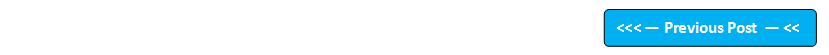## Blog Pages

### 49. Arithmetic OperatorsOperators in Java are just symbols used to perform operations on the provided data. Lets say we have an operator + , which is used to add values or values stored in variables.

Example -

1+2  //Here plus symbol is an operator which can be used to perform addition of two numbers.

Like this we have many operators which perform various operations. Lets learn them practically by following the current and few next posts.

How to use the Relational operators will be explained in the next post

Chandana said...

Hi Arun,
in the second program can't we use like this..may i know the reason why it is not possible

int a = 10.05+ 0.01
double b = (double) a

B Dinesh said...

in last example, result shouldn't be like below
1
1
1
1

Arun Motoori said...

@B Dinesh - You are wrong.

Arun Motoori said...

@Chandana - You will get compiler error as you cant assign a decimal value to an int data type declared variable.

Unknown said...

Hi Arun,

Can you explain the difference between the following two programs? I am bit confused between the outputs of both programs.

(1)
class PrefixVsPostfix
{
public static void main(String args[])
{
int a=1;
int b=2;
int c=++b; // Here the value of variable 'b' will get incremented first and then the incremented value gets assinged to vartiable 'c'
int d=a++; // Here the value of variable 'a' will get assigned to variable 'd' and later the value of variable 'a' gets incremented

System.out.println("a="+a);
System.out.println("b="+b);
System.out.println("c="+c);
System.out.println("d="+d);

}
}

a=2
b=3
c=3
d=1

(2)
class PrefixVsPostfix
{
public static void main(String args[])
{
int a=1;
int b=2;

System.out.println("a="+a);
System.out.println("b="+b);

int c=++b;
int d=a++;

System.out.println("c="+c);
System.out.println("d="+d);

}
}

a=1
b=2
c=3
d=2